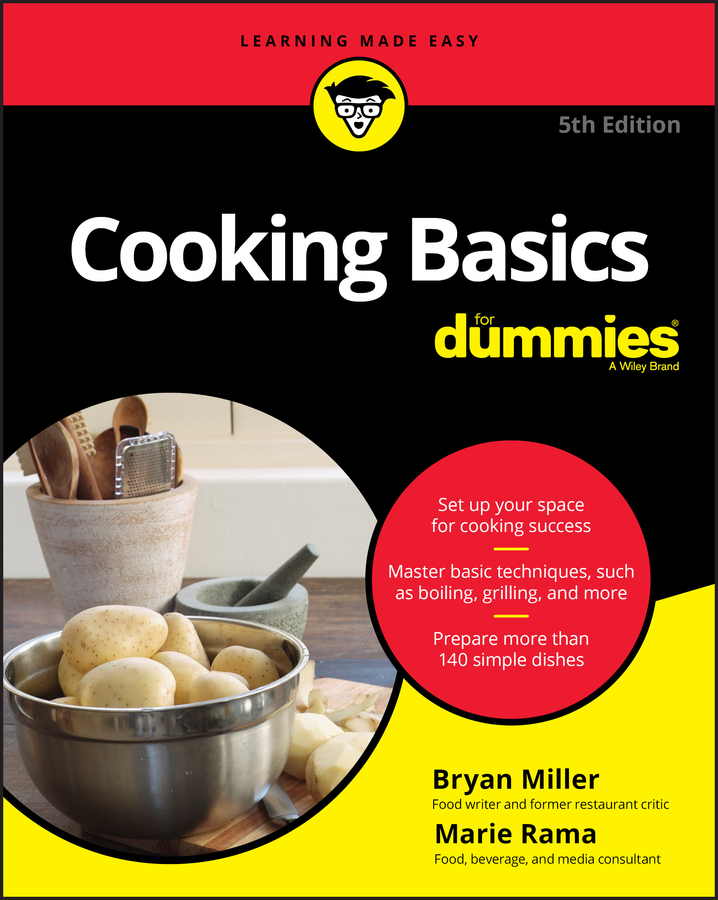##### Cooking Basics For DummiesPreparing lowfat dishes is easier when you know some basic cooking conversions. If you're math-challenged, don't worry. This list of common cooking conversions will help, especially if you don't have whole sets of measuring tools for cooking or if you need to know metric measures.

Note: Some metric equivalents are approximate.

• Temperature:

• Celsius = (°F – 32) ÷ 5/9

• Fahrenheit = (°C × 9/5) + 32

• Volume:

• 1 ounce = 28.35 grams

• 1 pound = 453.59 grams

• 1/2 tablespoon = 1-1/2 teaspoons = 7.5 milliliters

• 1 tablespoon = 3 teaspoons = 15 milliliters

• 2 tablespoons = 1 fluid ounce = 30 milliliters

• 4 tablespoons = 1/4 cup = 60 milliliters

• 5 tablespoons + 1 teaspoon = 1/3 cup = 75 milliliters

• 8 tablespoons = 1/2 cup = 120 milliliters

• 12 tablespoons = 3/4 cup = 180 milliliters

• 16 tablespoons = 1 cup = 8 fluid ounces = 240 milliliters

• 2 cups = 1 pint = 16 fluid ounces = 480 milliliters

• 2 pints = 1 quart = 32 fluid ounces = 1 liter

• 4 quarts = 1 gallon = 4 liters